# Why an antenna radiates

Home - Techniek - Electronica - Radiotechniek - Radio amateur bladen - QST - Why an antenna radiates

You don't have to know how an antenna works to use one, but getting a handle on this subject can deepen your understanding of radio. Here's a searching look at the mysterious process by which our antennas hurl energy from Here to There.

If antennas didn't radiate, we might be well advised to dispose of our ham gear and take up another hobby. Fortunately, they do radiate. Our purpose here is to explore the basic principles underlying this wonderful and little-understood phenomenon.

Before starting, let's try a simple test to see how much you already know about antennas. Don't peek at the answers(1) until you have answered all the questions!

 1 In a center-fed, half-wave dipole, electrons surge back and forth from one side of the antenna to the other. True____ False____ 2 It is possible for a perfect insulator to radiate. True____ False____ 3 Unlike ohmic resistance, "radiation resistance" has significance only at the feed point of an antenna or antenna system. True____ False____ 4 The ground around a transmitting antenna radiates. True____ False____

If you got all the answers right, you probably know more than the writer, and you have permission to skip the rest of this article.

### An imaginary experiment

Take a pithball about the size of a marble between your thumb and forefinger. If you don't know what a pithball is, or can't find one, a hunk of plastic foam or a ping pong ball will be fine. For lack of a better name we will refer to any of these articles as a pithball. Rub the pithball on your carpet to give it a good electrical charge. Now wave the pithball back and forth in the air over a six-inch distance as fast as you can. The pithball is sending out electromagnetic waves! Let's say you are achieving a rate of 10 cycles of motion per second. If you have placed in the corner of the room a sufficiently sensitive megameter-wave receiver, it will detect a signal when tuned to a frequency of 10 hertz, or to a wavelength of 30 million meters. If you could vibrate your hand fast enough you might even be able to carry on radio communication in this fashion!

We will continue this experiment shortly. Meanwhile let's take a look at what goes on inside an antenna.

### Put your antenna under a microscope

Now, admittedly the average antenna doesn't contain any pithballs. But it does contain hordes of minute, lightweight, electrically charged particles called electrons. Many of these are so-called free electrons that have broken loose from their parent copper or aluminum atoms and are able to travel more or less freely through the spaces between atoms, under the influence of any electric fields that may be present. The free electrons behave in many ways like tiny pithballs.

We know that an electric current in a conductor is simply a mass migration of free electrons. If the current is alternating, as in an antenna, the free electrons in a given locality move back and forth in unison. Evidently, then, any individual electron moves to and fro around an average posi tion, like the pithball in our experiment. Let's see how far and how fast this electron might travel.

Consider an antenna made of #12 copper wire and operating at 14.1 MHz. Each free electron near the surface of the wire is executing 14.1 million cycles of motion per second. Knowing the number of free electrons per cubic inch of copper, the electric charge on each, and the depth of RF penetration into the wire (the skin depth), we can calculate the peak speed of an electron at a place where the RMS antenna current is, say, one ampere. The result comes out to be less than half an inch per second. At that rate the electron doesn't move very far during each half cycle of vibration: its peak-to-peak travel is no more than a hundred-millionth of an inch. In the eyes of an electron this distance is quite respectable, being tens of thousands of times its own diameter. The answer to question 1 above, though, is clearly "False." Not one electron makes it through the feed system from one side of the antenna to the other.

We can compute the electron's deceleration and acceleration, which are greatest when the electron is coming to a stop and then starting up in the other direction. At an antenna current of one ampere, these quantities reach more than 50,000 gs! And an accelerating or decelerating charged body, be it an electron or a pithball, is a source of electromagnetic radiation.

A pithball is a pretty good insulator, and so, presumably, is an electron. So the answer to question 2 is "True": a perfect insulator can radiate.

Let's return to our imaginary experiment. This time, instead of waving a pithball at 10 hertz, we'll pretend to vibrate a single electron at radio frequency(2) and examine the resulting fields.

### Fields of a vibrating electron

The detailed structure of an electron is unknown, but for our purposes it doesn't matter; we can assume that our electron is a little round ball with an electrical charge distributed uniformly over its surface. We determine the fields by solving Maxwell's equations(3) at all distances from the electron, right down to its surface. This analysis is not an exercise for the faint-hearted. We'll skip the details and concentrate here on the results.

#### The Coulomb field

Grasping the electron in, say, a tiny pair of tweezers, let's start by holding it still. After a while the only field present will be a stationary electric field that points outward(4) in all directions from the electron. The field lines take the form illustrated in Fig 1 for both positive and negative charges. This is called the coulomb field. It is always present, regardless of whether the electron is in motion. We'll find later that the coulomb field plays a vital role in the operation of antennas.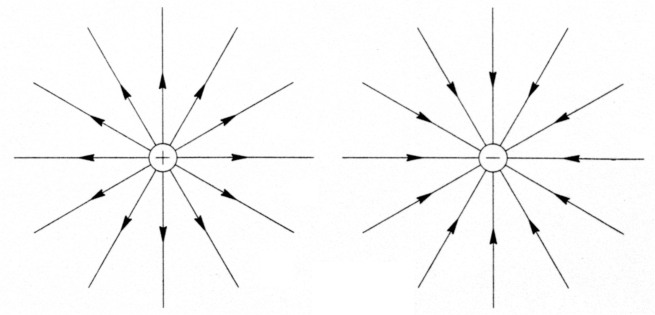Fig 1 - Always present around a charged particle, the coulomb field plays a vital role in energy radiation.

With vibratory motion, two new fields make their appearance.

#### The magnetic field

A moving electron constitutes a current, and a current is always surrounded by a magnetic field. As if hitchhiking, point the thumb of your right hand in the direction of the electron's motion; then your curled fingers represent the circular lines of the magnetic field around the electron. Point your thumb in the opposite direction and you see that the magnetic field reverses, so a vibrating electron gives rise to an alternating magnetic field. At the electron's surface, the magnetic field is almost exactly in phase with the electron's speed, but as we move away, the phase of the magnetic field begins to lag. Out to a radius of 1/6 wavelength, the phase lag is small. Beyond this radius, the lag starts to increase at a more rapid rate and soon settles at 360 degrees per wavelength of distance.

#### The dynamic electric field

The second new field is an electric field that results from the electron's acceleration. Because of its dynamic origin - in contrast to the electrostatic nature of the coulomb field - we'll call this field the dynamic electric field. It's useful to regard the dynamic electric field as the sum of two separate fields, one of which is in phase with the magnetic field and the other 90 degrees out of phase. We will call the in-phase component the radiation field and the out-of-phase component the induction field. It is the radiation field that carries energy from an antenna into the surrounding universe.

Fig 2 shows an area the size of a football field in which a lone electron, greatly mag nified, is being vibrated at 14.1 MHz. The figure is a snapshot of the electron's radiation field taken at an instant when the electron is at the center of its travel and moving to the right, as indicated by an arrow. The curved arrows show the direction and strength of the radiation field. The dashed circles represent spherical wavecrests on which the field is at a local maximum. As we go outward from a wavecrest, the field decreases to zero, then reverses and rises again to the next wavecrest. Like ripples in a cosmic pond, these spherical waves are expanding outward at the speed of light, 300 million meters per second.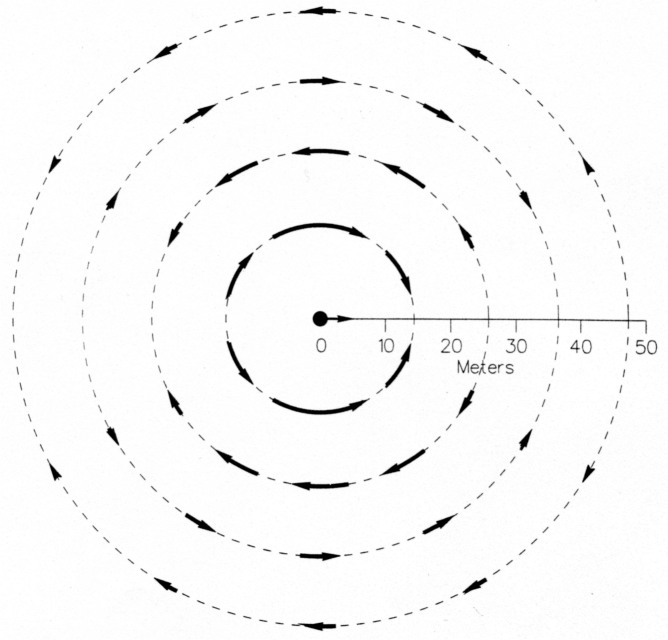Fig 2 - The radiation field of an electron vibrating at 14.1 MHz. Each broken line represents a wave crest.

At any one point, the radiation and induction fields vary as sine-wave functions of time. About 1/6 wavelength away from the electron (actually 1/[2π] wavelength), in a direction at right angles to the line of motion, the two fields are equal in amplitude. As we move farther away, the induction field falls off so much more rapidly than the radiation field that we soon have essentially nothing but the radiation field. Inside a vacuum-dielectric coaxial transmission line carrying power in one direction, the ratio of the electric to the magnetic field is equal to 377 ohms. In our pure radiation field, which carries power in the same manner even though there are no physical conductors present, this ratio is likewise equal to 377 ohms - a value that is sometimes called the characteristic impedance of space.

### Why an accelerating electron radiates

We've described the radiation field of a vibrating electron, but we haven't yet explained why it happens. The answer is hidden in Fig 3A.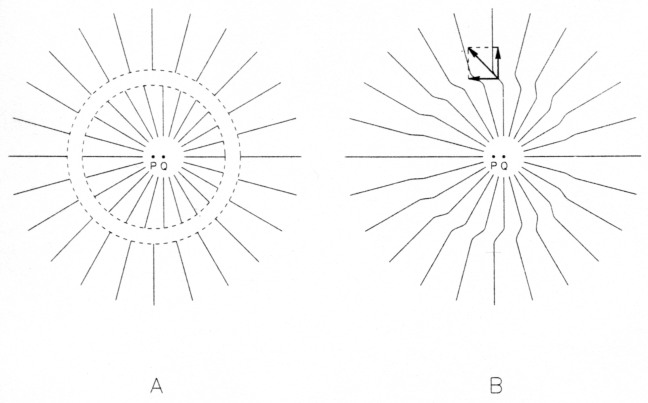Fig 3 - The electric field of an electron that was accelerated recently. The resulting disturbance in the field is traveling outward at the speed of light. The transverse component of the disturbed field is the radiation field.

Suppose that, until a short while ago, an electron was held at rest at point P in Fig 3A. It was then accelerated briefly to the right by our tweezers and afterward was kept moving to the right at constant speed. At the present time (which we'll call time zero), the electron is passing point Q.

Fig 3A contains two circles. The larger circle (the outermost broken line) is centered at P and has radius equal to the distance light would travel in the interval from the beginning of acceleration until time zero. The smaller circle is centered at the spot occupied by the electron at the end of acceleration; its radius is equal to the distance light would travel between the end of acceleration and time zero. As time marches on, the circles evidently grow at the speed of light. The space between the circles is equal to the distance light would travel during the period of acceleration. If the electron moves slowly in comparison with light, as it does in an antenna, the distance it covers during acceleration is small compared to the size of the circles, so the circles are nearly concentric. For clarity we have great ly exaggerated the distance PQ; it too would be very small if drawn to scale. Now we can determine what the electric field must look like at time zero.

Outside the larger circle, the field at time zero is a stationary coulomb field centered on P, as if the electron had never started to move.(5) Inside the smaller circle, the field is a moving coulomb field centered on the electron's present position, point Q. Between the circles the field is intermediate between the fields in the other two regions.

Now connect the field lines across the space between the circles and erase the circles, making Fig 3B. You can see that the electron, while accelerating, gave birth to an expanding electromagnetic disturbance. In the disturbed region, as shown by the arrows, there is a transverse field component - the radiation field - in addition to the outward-pointing coulomb field.

The radiation field resulting from a vibrating electron, Fig 2, is simply a continuous series of such disturbances caused by successive intervals of changing acceleration and deceleration.

### The bootstrap forces

The radiation and induction fields of a vibrating electron exist right down to the electron's surface. Since the electron's surface carries an electric charge, and since an electric charge is pulled by an electric field, it's fair to ask whether these fields are able to exert forces on the very electron that is producing them. In other words, can an electron "feel" its own dynamic electric field? The answer is yes. The electron is pulled by its own bootstraps! The tweezers that are providing the motive power must overcome the bootstrap forces.(6)

The bootstrap forces are responsible for two very important properties of a conduc tor: radiation resistance and inductance.

### Radiation resistance versus ohmic resistance

By our definition, an alternating radiation field is in phase with the accompanying magnetic field. At the surface of a vibrating electron the magnetic field is essentially in phase with the electron's speed, so here the radiation field, and the bootstrap force exerted by it, are likewise in phase with this speed. The direction of the force is such as to resist the electron's motion. It is evident that the force feels to our tweezers like a drag proportional to speed, as if the electron were moving through a viscous fluid. This drag force is the cause of radiation resistance.

An electron moving in a conductor also feels a drag force that is due to frequent progress-impeding collisions between the electron and the atoms in its path. This drag is the cause of ohmic resistance, the familiar R in Ohm's Law.

Both kinds of resistance dissipate energy at a rate equal to the resistance times the square of the current. Of course, energy dissipated this way doesn't actually disappear. An alternating current, flowing against radiation resistance, turns electrical energy into radiant energy, which wings its way off into space. Current flowing against ohmic resistance transforms electrical energy into heat, which is mechanical vibration of the atoms of the conductor - the atoms vibrate when they're hit by the moving free electrons.

Radiation resistance varies along the length of an antenna wire, but it is independent of the diameter and material of the conductor. The middle third of a half-wave, 14.1-MHz dipole has a radiation resistance of 3.7 ohms per foot. That's nearly 80 times the ohmic resistance of clean #12 copper wire at this frequency. Closer to the ends of the antenna, the radiation resistance is even higher.

### Inductance

At the surface of a vibrating electron, the induction field, being 90 degrees out of phase with the magnetic field, is 90 degrees out of phase with the electron's speed (ie, the current). The bootstrap force of the induction field therefore opposes the rate of change of current rather than the current itself. Here we see the underlying cause of the property of inductance. In reacting to this bootstrap force our tweezers deliver energy to the electron during acceleration and receive back an equal amount of energy from the electron during deceleration. The delivered energy is stored in the magnetic field around the moving electron and is returned when the magnetic field collapses as the electron slows down.

Because the bootstrap force of the induction field is proportional to acceleration, it feels to the tweezers just like mechanical inertia. In consequence the electron has an effective inertial mass that greatly exceeds its gravitational mass.

Now let's step back and look at the fields in and around an entire antenna. You will recognize that the basic principles involved apply to any kind of antenna. Because of its simplicity, we will use an isolated, center-fed, half-wave dipole as an example.

### The big picture

If our antenna doesn't contain any pith-balls, it doesn't contain any tweezers either. What is it, then, that causes the free electrons to vibrate? In real life it takes an electric field to move an electron. Inside an isolated straight dipole, the motive power comes from the combined coulomb fields of all the charged particles, positive and negative, in the antenna. We'll refer to this combined field as the antenna's coulomb field.

In addition to the coulomb field, the antenna as a whole exhibits a magnetic field that is the sum of the magnetic fields of all the moving free electrons. It also sports a dynamic electric field that is the vector sum of the dynamic electric fields of all the free electrons. As we did with an individual electron, we can separate the dynamic electric field of the antenna at any point in space into two components, one in phase with the total magnetic field and the other 90 degrees out of phase. We will call the in-phase component the radiation field of the antenna and the out-of-phase component the induction field. Right at the antenna, both fields are parallel to the metal surface.

It happens that the coulomb field and the induction field fall off much more rapidly than the radiation field with increasing distance from the antenna. At distances greater than a few wavelengths from the antenna, in what is called the antenna's far field, the electric field is essentially pure radiation.(7) Closer to the antenna, we have the near field, which is a mixture of the radiation, induction and coulomb fields.

### Action of the Coulomb field

We see in Fig 4 a snapshot of the coulomb field near the antenna. This picture shows an instant in time when the right-hand half of the antenna is positively charged and the left-hand half is negatively charged, as a result of a process that we'll examine in a moment. A half-cycle later, the polarity, and all the arrows, will be reversed. The spacing between the field lines indicates field strength. At the antenna wire or tubing, the field lines are nearly, but not exactly, perpendicular to the metal surface.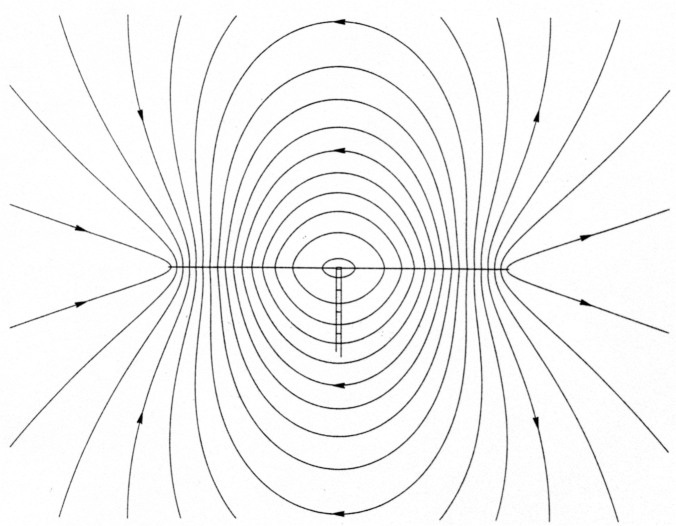Fig 4 - The coulomb field at an instant in time around a half-wave resonant dipole. A halfcycle later, the polarity, and all the arrows, will be reversed. The spacing between the field lines indicates field strength.

On alternating halves of the antenna, the perpendicular component of the coulomb field tries to pull electrons out of the surface. This effort is generally unsuccessful in amateur antennas because the "work function" for copper or aluminum - the energy it takes to dislodge an electron from the surface - is too great. If the transmit ter power is very high, though, the field may be strong enough to pull electrons out into the air. The result is an exciting (but power-wasting) luminous display called corona. But because the coulomb field leans slightly away from the perpendicular at the antenna's surface, it can always pull free electrons along the surface.

At one point in the RF cycle, free electrons throughout the antenna are moving to the right at or near their maximum speed. The right-hand half of the antenna thereupon begins to accumulate an excess of electrons, even if no single electron will shift to the right by more than a hundred-millionth of an inch. In the left-hand half of the antenna the departure of free electrons leaves an equal excess of oppositely charged metal ions, which are stationary atoms that have lost an electron. The coulomb field produced by this increasing imbalance of charges now opposes the electrons' rightward motion. By virtue of their effective mechanical inertia (also known as the bootstrap force of their induction field), the electrons coast for a while against the rising force of the coulomb field, which eventually brings them to a stop and then propels them back toward the left. After the electrons again reach maximum speed, now in the opposite direction, the foregoing scenario is repeated with left and right interchanged. The end result is the vibratory motion of free electrons that causes them to heat the metal and generate electromagnetic waves.

Newton's second law of motion tells us the relationship between the acceleration of an electron and the sum of the forces acting on it. In this case, one of the forces is the pull of the coulomb field parallel to the metal surface. The other two forces are the bootstrap force of the dynamic electric field and the drag of ohmic resistance. According to Sir Isaac, the sum of all three forces is equal to the gravitational mass of the electron times its acceleration. We will assume that an electron is so nearly weightless that its gravitational mass can be set to zero in this equation. Then the three forces must always add up to zero.

Turning this statement around another way, we can say that the dynamic electric field and the parallel component of the coulomb field partly cancel each other, the remaining field being just enough to overcome the drag of ohmic resistance. If the ohmic resistance is small enough to be ignored, the coulomb field is precisely equal and opposite to the dynamic electric field everywhere on the surface of an antenna.

This result leads to a procedure, which amounts to the construction and inversion of a matrix, for computing the current distribution on an antenna. Then, using the principles we've been discussing, we can compute the behavior of the system in detail.

### Power flow through space

Electrical engineers use the term real power for power that flows in one direction past a given point. They also speak of reactive power, which is power that flows back and forth in alternating directions with a net flow of zero in any one cycle. The radiation field of an antenna transmits only real power, which travels out toward distant localities without ever reversing direction. The induction field carries only reactive power, and the coulomb field carries both real and reactive power. Again we'll illustrate this with an isolated, center-fed, half-wave dipole.

#### Real power flow

How do you suppose your hard-earned RF power gets from the feed point to the rest of the antenna? You might think that it flows out through the wire or tubing of the dipole, but actually this real power is carried through the surrounding space by the coulomb field. Some lines of power flow are plotted in Fig 5.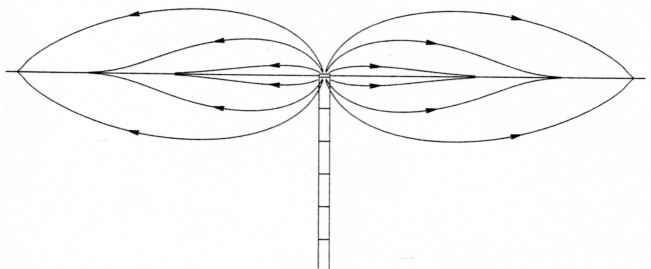Fig 5 - Flow of power from the feed point of a half-wave resonant dipole. The coulomb field around the antenna conductors carries power through the space surrounding the conductors.

As you see in the figure, when power coming up the feed line reaches the antenna it spews out into the air in all directions. It then arches to the right and left on curved paths that eventually intersect the antenna. At the points of intersection the coulomb field donates its real power to the free electrons, making up for the energy they are losing to ohmic resistance and radiation resistance.

#### Reactive power flow

During one interval in the RF cycle, the charge on the antenna reaches a maximum, and the current and the magnetic field go through zero. A quarter of a cycle later, the reverse is true. In the first interval, the antenna is surrounded by a cloud of electrostatic energy stored in the coulomb field. In the second interval, the coulomb field has disappeared, and we find the same energy stored in the magnetic field. The energy stored in the coulomb field is used in accelerating the effective inertial mass of the free electrons, which by their motion create the rising magnetic field. Energy thus moves from the coulomb field, via the induction field, to the magnetic field, only to move back again during the next quarter cycle as the magnetic field collapses. This is reactive power flow, with a net of zero.

You can think of the cloud of electrostatic energy as energy stored in the distributed capacitance between the two halves of the antenna. Similarly, the stored magnetic field energy can be thought of as energy residing in the distributed inductance of the antenna wire. If power is suddenly cut off by short-circuiting the feed point, the antenna doesn't stop radiating right away. Instead, it oscillates with diminishing vigor at its resonant frequency until the energy stored in the fields has been dissipated in ohmic resistance and radiation resistance. Our antenna can be accurately described as a resonant circuit made up of distributed capacitance, distributed inductance, and two kinds of distributed resistance.

### Antennas and non-antennas

What do the following items have in common: a dipole antenna, a radar dish, the ground around a transmitting antenna, a coil and capacitor in parallel, and a wire carrying music to a loudspeaker? One answer: to a greater or lesser degree, they all radiate!

This is true because in operation they all carry time-varying currents and, consequently, accelerating electrons. The dipole antenna is an example of a distributed circuit that owes its existence to the fact that it radiates well. It is designed for efficient conversion of electrical energy into radio waves. But any system of conductors that carries varying currents behaves in accordance with the principles described earlier. The same processes, including radiation, take place whether we call the system an antenna or something else. For example, what we generally refer to as reflection from a conducting surface (the radar dish, the ground) is actually radiation from free electrons set in motion by incident electric fields.

### In sum

Perhaps the foregoing intuitive introduction to classical electromagnetic theory will inspire further development and refinement of the ideas presented. In any event, I hope you'll be able to contemplate an antenna, or a non-antenna, with a warm feeling for all the interesting things going on in and around it!

### Notes

1. 1 - False. 2 - True. 3 - False. 4 - True.
2. The classical electromagnetic theory we use in this article is valid at frequencies from dc through microwaves. At still higher frequencies one must venture into the realm of quantum electrodynamics.
3. For some pertinent theoretical background see Feynman, Leighton and Sands, The Feynman Lectures on Physics, Vol II (Addison-Wesley Publishing Co, 1964).
4. To get an outward-pointing field we must take the charge on the electron to be positive instead of negative as is actually the case. I find it easier to visualize the interaction of electric fields and electrons by thinking of the electron charge as positive. In the end it doesn't make the slightest difference. After all, Ben Franklin, on rubbing an ebony rod with cat's fur, could just as well have defined the charge induced on the cat to be positive. Life might be simpler today if he had.
5. If you were observing from anywhere outside the larger circle at time zero, the electron would appear to be at point P instead of its actual position, point Q. That's because, according to Einstein's theory of relativity, the news that the electron has started to move can't propagate faster than the speed of light. If you were anywhere inside the smaller circle, the electron would appear at time zero to be in its actual position.
6. The electron is pulled by its own coulomb field too, but equally in all directions, so the net effect is zero.
7. In summing the radiation fields at a given point in the far-field region from all the free electrons, we must take into account the differing phases of the source electrons and the varying propagation times. The resulting phase variations cause partial reinforcement or cancellation of the fields, to a degree that depends on direction from the antenna. This effect is the basis of the very extensive subject of antenna radiation patterns, which we will not discuss further here.

W7TX, Kenneth Macleish.